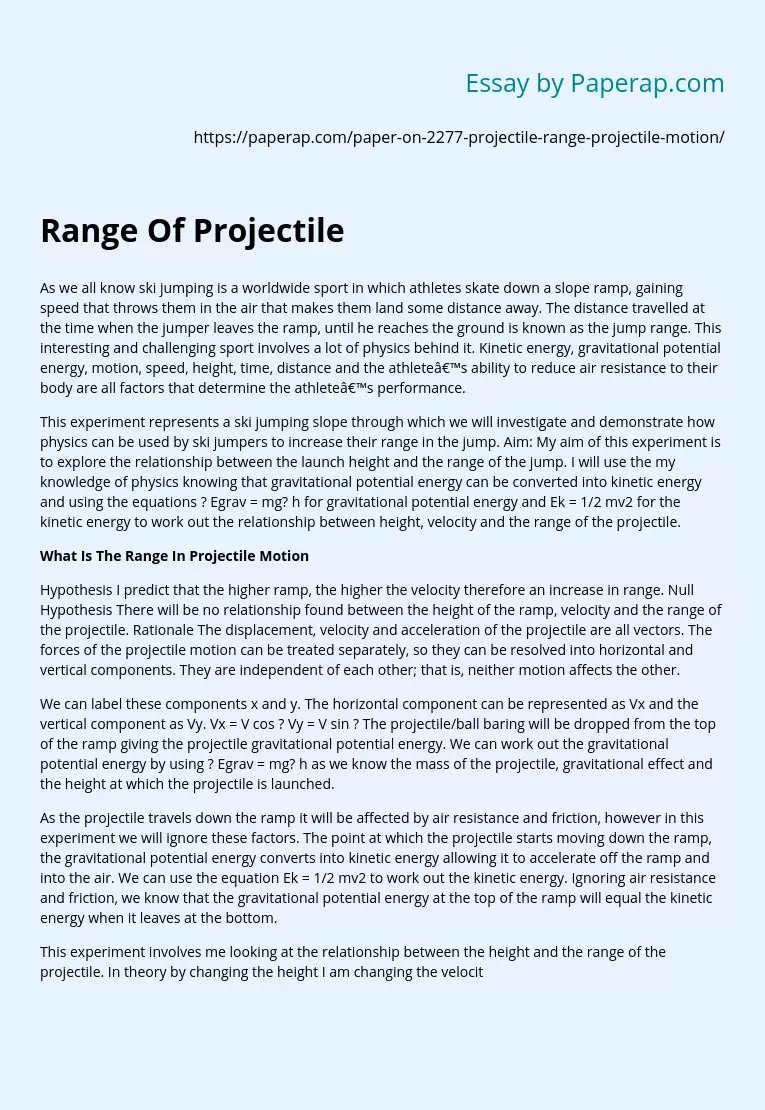# Range Of Projectile

As we all know ski jumping is a worldwide sport in which athletes skate down a slope ramp, gaining speed that throws them in the air that makes them land some distance away. The distance travelled at the time when the jumper leaves the ramp, until he reaches the ground is known as the jump range. This interesting and challenging sport involves a lot of physics behind it. Kinetic energy, gravitational potential energy, motion, speed, height, time, distance and the athlete’s ability to reduce air resistance to their body are all factors that determine the athlete’s performance.

This experiment represents a ski jumping slope through which we will investigate and demonstrate how physics can be used by ski jumpers to increase their range in the jump. Aim: My aim of this experiment is to explore the relationship between the launch height and the range of the jump. I will use the my knowledge of physics knowing that gravitational potential energy can be converted into kinetic energy and using the equations ? Egrav = mg? h for gravitational potential energy and Ek = 1/2 mv2 for the kinetic energy to work out the relationship between height, velocity and the range of the projectile.

## What Is The Range In Projectile Motion

Hypothesis I predict that the higher ramp, the higher the velocity therefore an increase in range. Null Hypothesis There will be no relationship found between the height of the ramp, velocity and the range of the projectile. Rationale The displacement, velocity and acceleration of the projectile are all vectors.

Get quality help nowBella HamiltonVerified

Proficient in: Energy5 (234)

“ Very organized ,I enjoyed and Loved every bit of our professional interaction ”+84 relevant experts are online

The forces of the projectile motion can be treated separately, so they can be resolved into horizontal and vertical components. They are independent of each other; that is, neither motion affects the other.

We can label these components x and y. The horizontal component can be represented as Vx and the vertical component as Vy. Vx = V cos ? Vy = V sin ? The projectile/ball baring will be dropped from the top of the ramp giving the projectile gravitational potential energy. We can work out the gravitational potential energy by using ? Egrav = mg? h as we know the mass of the projectile, gravitational effect and the height at which the projectile is launched.

As the projectile travels down the ramp it will be affected by air resistance and friction, however in this experiment we will ignore these factors. The point at which the projectile starts moving down the ramp, the gravitational potential energy converts into kinetic energy allowing it to accelerate off the ramp and into the air. We can use the equation Ek = 1/2 mv2 to work out the kinetic energy. Ignoring air resistance and friction, we know that the gravitational potential energy at the top of the ramp will equal the kinetic energy when it leaves at the bottom.

This experiment involves me looking at the relationship between the height and the range of the projectile. In theory by changing the height I am changing the velocity of the projectile and so therefore the range will be affected. By re-arranging the equation Ek = 1/2 mv2 we can work out the velocity of the ball baring, 2Ek/m = v2 then do V v2 = v. This can also be simplified to give V (2mgh) /m = v. The “m” cancels which will leave us with V = V (2gh). We can then use the equation V = Distance/Time which will give the theoretical horizontal value.

We can re-arrange this equation to give us the distance, Distance = Velocity x Time. This is the horizontal component. Changing the vertical height of the drop from the ramp would vary the range. This is because according to the law of energy, it cannot be made or destroyed but transferred. To apply this law into this practical, we can say all of the gravitational potential energy is converted into kinetic energy assuming no energy is wasted. As a result, gravitational portential energy is equal to kinetic energy.